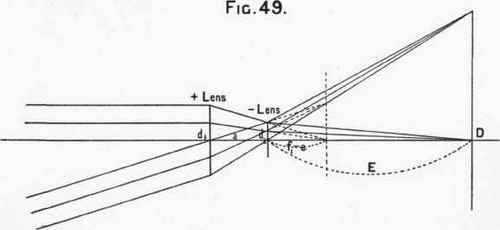Scale, 3/4.

Fig. 48* exhibits at a glance the meaning of a "rapid" as distinguished from a "slow" lens. For convenience we have illustrated a lens of 4 inches focal length, but it is evident that the cones of light will be of the same shape, or the rays will cross at the same angle, for any given intensity, no matter what the focal length of the lens may be. The figure also shows that rapid lenses have less "depth of focus" than slow lenses, but we shall refer to this further on.

In designing a Telephotographic combination we must carefully consider the purpose for which it will be employed. If we take a positive lens of high intensity we can convert it into a Telephotographic system by combining it with a negative lens, which may be destined for either rapid work, or, on the other hand, to attain high magnification. For example, if we take a positive lens, having an intensity of f/4 we can employ a negative lens to magnify the image three times, and yet still have a fairly rapid lens in the combination, of intensity f/12. Again, if high magnification is the aim, we can magnify the image given by the positive lens as much as sixteen times and the combination still has a reasonable intensity of f/64.

On the other hand, if we take a positive lens of only moderate intensity, say f/8, and combine it with a negative lens, we can only expect to get small or moderate amplifications without necessitating a very low intensity in the Telephotographic combination.

The Lowest Intensity Permissible. - In treating of diffraction, Lord

* From "A Simple Guide to the Choice of a Lens." (J. H. Dallmeyer, Ltd.) By the Author.

Rayleigh has demonstrated that the lowest intensity permissible without introducing the disturbing effects of diffraction is a ratio of aperture to focal length of 1: 71, or f/'71.

This conclusion has a very important bearing on the use of the Telephotographic lens. Suppose the intensity of the positive element is f/8 for example, and we wish to produce a magnification of five times, the combination will have an initial maximum intensity of f/40. We might very likely stop down the positive lens to f/16, or even less, with the object of getting finer definition. If the stop f/16 in the positive lens were employed, we should convert the intensity of the whole system into f/780, which is in reality too small for the finest definition.

We must then always remember that: the intensity of the positive element of the system divided by the magnification must not be less than f /71.

On Covering Power, or the size of plate covered at a given intensity. Referring to Fig. 49, the diameter of the circle beyond which no light can pass being called d, the effective aperture of the positive lens d1 and the diameter of the negative lens d2, and, as before, f1, vf2, represent their focal lengths, a the separation, and e the camera extension from the negative lens, it can be shown that:Approximate formula for maximum circle of illuminationIn the example shown in Fig. 43 d1 = 7/8", d2 = 5/4",/ = 6", f2 = 3", and e = 9 inches. HenceThese formulae only require a knowledge of arithmetic to arrive at the result. The result by the approximation, 10 1/8, differs very slightly from that of the more complicated formula, which gives 10 1/10 as the diameter of the field.

If we employ a diaphragm and so reduce the effective aperture of dl we must substitute its value in the formula to find the circle of illumination covered, or the diameter of the field. Say its effective aperture is 1/2 inch, then d = 8 inches.

In the formula it is evident that d1f2 may become very small when the effective aperture d1 is also very small.If we neglect this quantity we find the minimum circle of illumination for any lens

= (M - I) ( f1d2/f1-f2), where m is the magnification as before. In the case under notice d min. = (4 - 1)6x5/4------= 7 1/2 inches.

6- 3

The circles of illumination must determine the size of the plate that can be covered; the diagonal of the plate must not be more than the diameter of the circle covered, or the corners of the plate will be "cut off," or not illuminated. It is advisable to arrange that the circle of illumination is greater than the diagonal of the plate for the smallest effective aperture likely to be used. We give here the diagonals of the sizes of plates in ordinary use.

 Size of plate. 4 1/4x 3 1/4 5X4 6 1/2 x 4 3/4 8 1/2 x 6 1/2 10 x 8 12 x 10 15 x 12 18 x 16 22 x 20 25 X 21 30 x 24
 Diagonal or minimum diameter of circle of illumination. 5.4" 6.4 8 10.7 12.8 15.6 19.2 24.1 29.7 32.7 38.4

On the angle included by the combination.

Calling a the extreme angle, f the focal length of the Telephoto-graphic lens, and d the diameter of the circle of illumination, a = 2 tan - 1 D/2F .

In the case illustrated d= io. 1 and f=24 ;

a = 2 tan-1 10.1/48 = 2 tan-1 .210416.

From a table of natural tangents we find that .210416 corresponds to an angle of 11° 53', and hence the extreme angle that can be included is 230 46'. As the effective aperture of the diaphragm is decreased the diameter of the circle of illumination becomes less, or less angle is included as we stop down the lens.